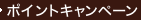•### Physics Laboratory Manual （3 LAB）

• ただいまウェブストアではご注文を受け付けておりません。 ⇒古書を探す
• 製本 Paperback:紙装版/ペーパーバック版／ページ数 494 p.
• 言語 ENG,ENG
• 商品コード 9780495114529
• DDC分類 530

### Full Description

Designed to help you develop your intuitive abilities in physics, the PHYSICS LAB MANUAL emphasizes conceptual understanding and includes a thorough discussion of physical theory to help you see the connection between the lab and the lecture. Each lab includes a set of pre-lab exercises, and many labs give you hands-on experience with statistical analysis.

### Table of Contents

`Preface                                            xiAcknowledgements                                   xiiiGeneral Laboratory Information                     1   (12)    Laboratory 1. Measurement of Length            13  (10)    Laboratory 2. Measurement of Density           23  (10)    Laboratory 3. Force Table and Vector           33  (10)    Addition of Forces    Laboratory 4. Uniformly Accelerated Motion     43  (10)    Laboratory 4A. Uniformly Accelerated Motion    Using a Photogate    Laboratory 5. Uniformly Accelerated Motion     53  (10)    on the Air Table    Laboratory 6. Kinematics in Two Dimensions     63  (10)    on the Air Table    Laboratory 7. Coefficient of Friction          73  (12)    Laboratory 7A. Coefficient of Friction    Using a Force Sensor and a Motion Sensor    Laboratory 8. Newton's Second Law on the       85  (10)    Air Table    Laboratory 9. Newton's Second Law on the       95  (10)    Atwood Machine    Laboratory 10. Torques and Rotational          105 (12)    Equilibrium of a Rigid Body    Laboratory 11. Conservation of Energy on       117 (10)    the Air Table    Laboratory 12. Conservation of Spring and      127 (10)    Gravitational Potential Energy    Laboratory 12A Energy Variations of a Mass    on a Spring Using a Motion Sensor    Laboratory 13. The Ballistic Pendulum and      137 (12)    Projectile Motion    Laboratory 14. Conservation of Momentum on     149 (10)    the Air Track    Laboratory 14A. Conservation of Momentum    Using Motion Sensors    Laboratory 15. Conservation of Momentum on     159 (10)    the Air Table    Laboratory 16. Centripetal Acceleration of     169 (10)    an Object in Circular Motion    Laboratory 17. Moment of Inertia and           179 (10)    Rotational Motion    Laboratory 18. Archimedes' Principle           189 (8)    Laboratory 19. The Pendulum---Approximate      197 (10)    Simple Harmonic Motion    Laboratory 20. Simple Harmonic                 207 (10)    Motion---Mass on a Spring    Laboratory 20A. Simple Harmonic    Motion---Mass on a Spring Using a Motion    Sensor    Laboratory 21. Standing Waves on a String      217 (8)    Laboratory 22. Speed of Sound---Resonance      225 (10)    Tube    Laboratory 23. Specific Heat of Metals         235 (8)    Laboratory 24. Linear Thermal Expansion        243 (8)    Laboratory 25. The Ideal Gas Law               251 (8)    Laboratory 26. Equipotentials and Electric     259 (10)    Fields    Laboratory 27. Capacitance Measurement with    269 (10)    a Ballistic Galvanometer    Laboratory 28. Measurement of Electrical       279 (10)    Resistance and Ohm's Law    Laboratory 29. Wheatstone Bridge               289 (10)    Laboratory 30. Bridge Measurement of           299 (8)    Capacitance    Laboratory 31. Voltmeters and Ammeters         307 (12)    Laboratory 32. Potentiometer and Voltmeter     319 (10)    Measurements of the emf of a Dry Cell    Laboratory 33. The RC Time Constant            329 (10)    Laboratory 33A. RC Time Constant with    Positive Square Wave and Voltage Sensors    Laboratory 34. Kirchhoff's Rules               339 (10)    Laboratory 35. Magnetic Induction of a         349 (10)    Current Carrying Long Straight Wire    Laboratory 35A. Magnetic Induction of a    Solenoid    Laboratory 36. Alternating Current LR          359 (10)    Circuits    Laboratory 36A. Direct Current LR Circuits    Laboratory 37. Alternating Current RC and      369 (10)    LCR Circuits    Laboratory 38. Oscilloscope Measurements       379 (12)    Laboratory 39. Joule Heating of a Resistor     391 (10)    Laboratory 40. Reflection and Refraction       401 (12)    with the Ray Box    Laboratory 41. Focal Length of Lenses          413 (8)    Laboratory 42. Diffraction Grating             421 (10)    Measurement of the Wavelength of Light    Laboratory 42A. Single-Slit Diffraction and    Double-Slit Interference of Light    Laboratory 43. Bohr Theory of                  431 (10)    Hydrogen---The Rydberg Constant    Laboratory 43A. Light Intensity versus    Distance with a Light Sensor    Laboratory 44. Simulated Radioactive Decay     441 (10)    Using Dice ``Nuclei''    Laboratory 45. Geiger Counter Measurement      451 (12)    of the Half-Life of 137Ba    Laboratory 46. Nuclear Counting Statistics     463 (10)    Laboratory 47. Absorption of Beta and Gamma    473 (10)    RaysAppendix I                                         483 (2)Appendix II                                        485 (2)Appendix III                                       487`